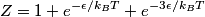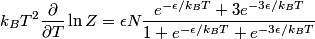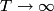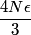## Solution to 1986 Problem 67

 The canonical partition function\begin{align*}Z = 1 + e^{-\epsilon/k_B T} + e^{-3\epsilon/k_B T}\end{align*}Therefore, the totaly energy of each particle is\begin{align*}k_B T^2 \frac{\partial}{\partial T} \ln Z &= \epsilon N \frac{e^{-\epsilon/k_B T} + 3 e^{-3 \epsilon/k_B T}...As$T \to \infty$, this goes to\begin{align*}\frac{4 N \epsilon}{3}\end{align*}Therefore, answer (C) is correct.